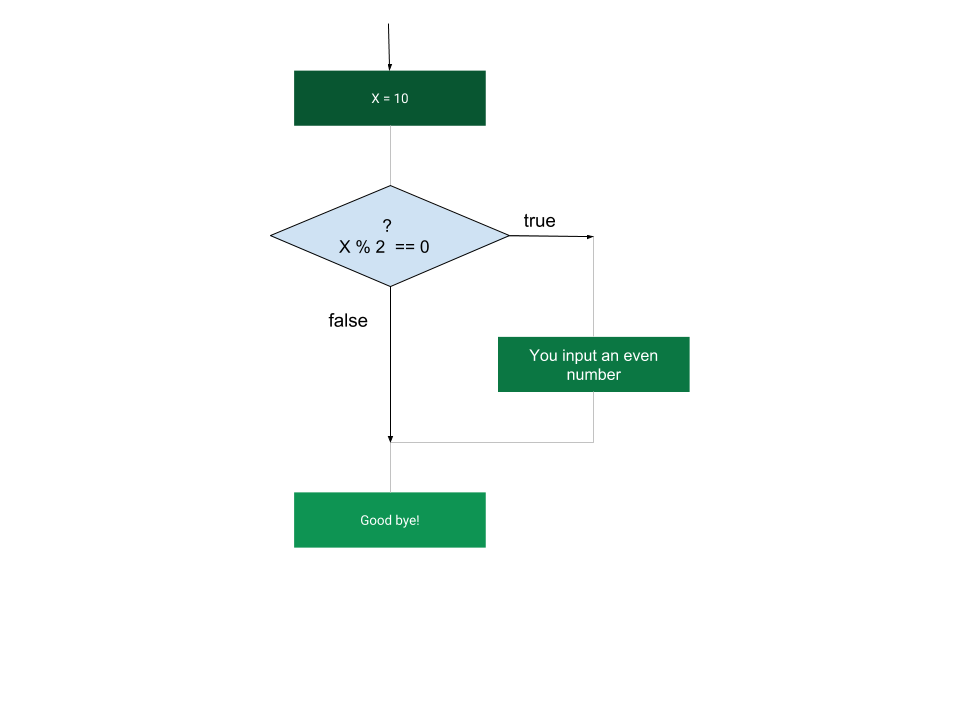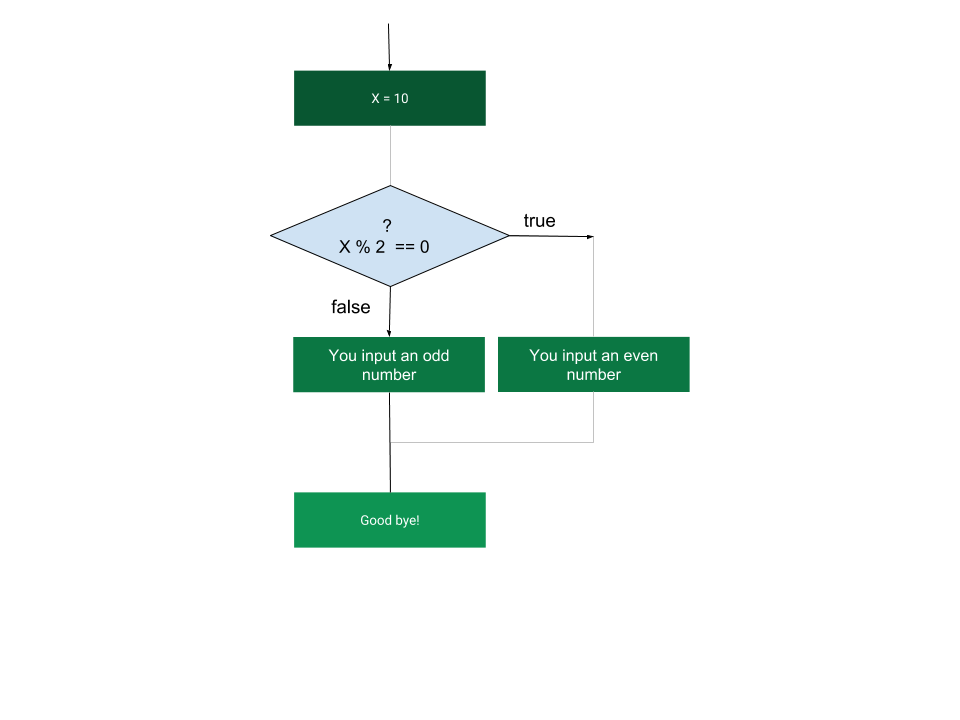# Compound Statements

So far you studied simple statements in Python. A simple statement consisted of one single statement which had no logic control relation to other statements before or after that statement. Simple statements are stand alone statements which are syntactically correct to use individually. When your program consists of only simple statements, the interpreter runs the statements one at a time in a sequence and once all the simple statements are executed, the program exits. However, you can change this sequential pattern of execution using Control Statements.

# Control Statements

Control Statements provide mechanisms to control the execution of a program based on certain conditions and thereby deviate from the sequential order. Python provides conditional if statement and loop structures - while and for as part of Control Statements.

Control Statements become part of a compound statement and should all be executed together based on the control statement's conditional result.

### Compound Statement

A compound statement contain groups of statements which all go together to form the compound statement. They span multiple lines and will generally have indentation which marks the groups to be executed as one block together. Although you may write a compound statement, all on the same line, without indentation, it is recommended to use indentation. When you do use the indentation, Python is strict about the number of indents you use relative to its predecessor statement. If you do not indent correctly you get IndentationError

Although there are many varieties of Compound Statements, in this chapter you will learn on one such statement which is the 'if', 'elif' and 'else'

## Conditional if statement

A very common conditional that is used in almost all programming languages is an if statement.

Before you proceed further on if let us understand the relational and logical operators available which are used in if and while statements.

The following are the relational operators in Python which all evaluates to True or False based on the expression. The two operands 9 and 8 shown below can be replaced by any expression consisting of one or more variables.

Operator name Notation Examples Result
Greater than > 9 > 8
'b' > 'a'
True
Less than < 9 < 8
'ba' < 'ab'
False
Greater than or equal to >= 9 >= 8
'ab' >= 'aa'
True
Less than or equal to <= 9 <= 8
'ab'<='ba'
False
Equal to == 9 == 8
'aa' == 'ba'
False
Not equal to != 9 != 8
'ba' != 'ab'
True

For strings it uses lexicographical ordering in evaluating the expressions as long as both operands are strings otherwise you get an error when you compare a string with a numerical value. Refer:http://docs.python.org/reference/expressions.html

The following are the logical operators in Python

Operator name Notation Example Result Comment
logical AND and
9 > 8 and 5 > 4
True Returns True if both expressions on either side of and evaluate to True. This operator evaluates the second expression only if the first expression evaluates to True
logical OR or 8 > 9 or 5 > 4 True Returns True if either one of the expressions on either side of or evaluate to True. This operator evaluates the second expression only if the first expression evaluates to False
logical NOT not not 9 > 8 False Reverses the value of the Boolean expression

### Operator Precedence

Here is the table showing the precedence order of all the operators in JavaScript including the Arithmetic operators. Highest precedence is on the top, lowest at the bottom.

Operator Order of Evaluation Description
not R -> L Unary operators
*, /, % L -> R Multiplication, division, modulo
+, — L -> R Addition, subtraction
<, <=, >, >= L -> R Relational operators
==, != L -> R Relational equality/identity operators
and, or L -> R Logical operators
=, +=, -= R -> L Assignment operators

To change the above order of precedence, you can place parentheses '()' around the expressions that should be evaluated first. In this case the innermost set of parentheses is evaluated first. This mostly works as intended except when the parenthesis is used with logical operators 'and' and 'or'. For example the below expression does not evaluate the parenthesis first as the expression to the left of logical operator is evaluated first.

`9>10 and (a>b)`

### Short-circuit concept

Logical operators 'and' and 'or' are also called short-circuit operators as it only evaluates the expression that is on the right of the operator after it figures out evaluating the left expression is not enough to decide the final result. In case of 'and' the right expression is evaluated, if the left expression evaluates to true. Because if the left expression is 'False', then the entire expresion will be false irrespective of the outcome of the right side of the expression. In that case, the evaluation of the right side of the operator is aborted as there is no point is finding that out. But if the left expression is 'True', then it proceeds further and evaluates the expression to the right of the operator.

In case of the 'or' operator, the right one is evaluated only when the left expression evaluates to 'False'. Based on similar principles, there is no point in evaluating the right side of the operator if the left side evaluates to 'True' as the entire expresion will be 'True' anyways irrespective of the result of the right side of the operator.

#### If Conditional Flowchart

The following flowchart depicts the control flow of the program based on the result of the boolean expression

x % 2 == 0

If 'x' is an even number then the `x % 2 == 0` return True, in which case the program enters the block of code which prints out 'You input an even number' and then 'Good bye'. If the conditional evaluates to False, then the program skips the block of code which prints 'You input an even number' and outputs only the 'Good bye'. So the program instead of going in a sequential order, takes a deviation based on a conditional expression.### The if Statement:

if (conditional expression which evaluates to True, False or any other literal value) :

_conditional expression is made up of one or more logical and relational expressions including combinations of both types which, on the whole, evaluates to True or False.

Note: You can also use arithmetic expressions or variables which evaluate to any literal value. If the expression evaluates to None or '0' then it is treated as False. Any other literal value is considered True_

A colon ':' should follow immediately after the conditional expression. If the conditional expression evaluates to True, then the program of execution starts from the indented statements after the : If the conditional evaluates to False then the program of execution skips the indented block after : and executes the statements after the if block.

The code for the above flowchart

``````
x = int(input())
if(x%2 == 0):
print("You input an even number")
print("statement after the if block")
``````

### The if...else Statement:

An if statement can be followed by an optional else statement, which executes when the boolean expression is false.

The syntax of an if...else is:

if (boolean_expression) :
//Executes when the Boolean expression is true
else :
//Executes when the Boolean expression is false

Run the above code and input an even and odd number and study how the if or else block gets executed based on boolean expression evaluation. The same execution can be visualized with the flow chart below.else is optional in an if statement.

### The if...elif...else Statement:

An if - else statement can be followed by an optional elif...else statements. When using if , elif , else statements there are few points to keep in mind.

• An if can have zero or one else and it must come after any if's.
• An if can have zero to many elif's and they must come before the final else.
• Once an elif succeeds, none of the remaining elif's or the final else's will be tested.

Now let us take a grading calculation as an example below to use the if- elif-else operators:

``````score = float(input("Please enter your score: "))
if score > 90:
print("Congratulations! you scored an 'A' grade")
elif score > 80:
print("Congratulations! you scored a 'B' grade")
elif score > 70:
print("Congratulations you scored a 'C' grade")
else:
print("Sorry you failed.")
``````

In the above program, you first get the input from the user, and then evaluate the first conditional if (score > 90) which is of the form

if (conditional expression which evaluates to True or False) :

If the conditional expression evaluates to True, then the program of execution starts from the indented statements after the : If the conditional evaluates to False then the program of execution skips the indented block after : and proceeds to elif statement and checks for conditional again. The same logic is applied on all other conditionals all the way till the else statement. The last else statement is executed if all conditionals expression above evaluated to False.

### Question

In the above conditional is it important to keep the first conditional as score > 90? If you change the order and instead have score > 70 as the first conditional, then will it still provide the desired functionality? Why or Why not?

### Short Hand If Notation

If you have only one statement to execute, you can put it on the one line.

``````
a = 3
b = 2
if b > a: print("b is greater than a")
``````

### Short Hand If-Else

Similarly, if you have only one statement to execute, one for if, and one for else, you can put it all on one line.

``````a = 3
b = 2
print (a) if b > a else print(b)
``````

Note there are no colon's used in these notations and this only works when the conditional has to execute just one line of code based on the conditional

### Short Hand Multiple if-else

``````a = 3
b = 3
print(a) if a > b else print('equals') if a == b else print(b)
``````

### Math module

For mathematical calculations, we can use the Math module that is already present in Python API. To use that we first have to import it. Here is an example to find the square root of a number using the Math module

``````
# import the math module
import math

math.sqrt(4)
``````

Note: A module is nothing but a software program which is packaged and distributed so that others can use it. You will learn more on modules in Chapter 6

While the math module provides methods to work on floating point numbers, you can use cmath module to work with imaginary (complex) numbers. So if you try to find square root of -1 using math module, it raises an error but instead if you use cmath then you will get 1j as the result.